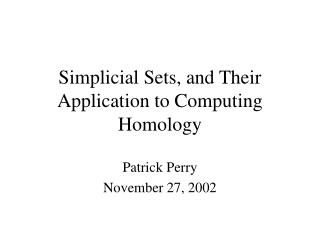DownloadDownload PresentationSimplicial Sets, and Their Application to Computing Homology

# Simplicial Sets, and Their Application to Computing Homology

Télécharger la présentation## Simplicial Sets, and Their Application to Computing Homology

- - - - - - - - - - - - - - - - - - - - - - - - - - - E N D - - - - - - - - - - - - - - - - - - - - - - - - - - -
##### Presentation Transcript

1. Simplicial Sets, and Their Application to Computing Homology Patrick Perry November 27, 2002

2. Simplicial Sets: An Overview • A less restrictive framework for representing a topological space • Combinatorial Structure • Can be derived from a simplicial complex • Makes topological simplification easier • Possibly a good algorithm for Homology computation

3. Motivation • If X is a topological space, and A is a contractible subspace of X, then the quotient map X  X/A is a homotopy equivalence • Any n-simplex of a simplicial complex is contractible

4. Example Simplification

5. Another Simplification

6. Geometry Is Not Preserved • Collapsing a simplex to a point distorts the geometry • After a series of topological simplifications, a complex may have drastically different geometry • Does not matter for homology computation

7. Cannot use a Simplicial Complex! • Bizarre simplices arrise: face with no edges, edge bounded by only one point • Need a new object to represent these pseudo-simplices • Need supporting theory to justify the representation

8. Simplicial Sets • A Simplicial Set is a sequence of sets K = { K0, K1, …, Kn, …}, together with functions di : Kn Kn-1 si : Kn Kn+1 for each 0  i  n

9. Simplicial Identities • didk = dk-1di for i < k • disk = sk-1di for i < k = identity for i = j, j+1 = skdi-1 for i > k + 1 • sisk = sk+1si for i  k

10. Simplicial Complexes as Simplicial Sets • A simplicial set can be constructed from a simplicial complex as follows: Order the vertices of the complex. Kn = { n-simplices } di = delete vertex in position i si = repeat vertex in position i

11. Homology of Simplicial Set • Chain complexes are the free abelian groups on the n-simplices • Boundary operator:    (-1)i di • Degenerate (x = si y) complexes are 0 • Homology of Simplicial Set is the same as the homology of the simplicial complex

12. Bizarre Simplices are OK • Simplicial sets allow us to have an n-simplex with fewer faces than an n-simplex from a simplicial complex • Our bizarre collapses make sense in the Simplicial Set world

13. What has Trivial Homology?

14. Example From Before Makes Sense

15. New Example: Torus

16. End Result for Torus • We have eliminated 8 faces, 16 edges, and 8 vertices • Cannot simplify any further without affecting homology

17. Benefit of Simplicial Set • More flexibility in what we are allowed to do to a complex • Linear-time algorithm to reduce the size of a complex • Can use Gaussian Elimination to compute Homology of simplified complex

18. Can We Simplify Further? • What about (X  X/A) + bookkeeping?

19. Bookkeeping • Using Long Exact Sequence, we can figure out how to simplify further: d(Hn(X)) = d(Hn(A)) + d(Hn(X/A)) + d(ker in-1*) - d(ker in*) • If i* is injective, bookkeeping is easy

20. Torus (Revisited)

21. Collapsing the Torus to a Point • Inclusion map on Homology is injecive in each simplification •  = (0, 0, 0) + (0, 1, 0) + (0, 1, 0) + (0, 0, 1) = (0, 2, 1)

22. Good News • Computation of ker i* is local • Potentially compute homology in O(n TIME(ker i* ))

23. Conclusion • A less restrictive combinatorial framework for representing a topological space • Can be derived from a simplicial complex • Makes topological simplification easier • Possibly a good algorithm for Homology computation